## Fibonacci Trading Strategy – Complete Tutorial

• What Fibonacci retracement levels should I use?
• Should the price touch the retracement levels?
• What confluence factor to check for more reliable entry?
• How to take entry (aggressive /conservative/safe entry)?
• Where to put the stop loss-based Fibonacci retracement ratio?
• Where to take profit based on Fibonacci?
• Trailing stop loss and profit booking method based on the Fibonacci extension tool

You will find some answers to the following common question related to Fibonacci trading

• Does Fibonacci work in trading?
• How successful is Fibonacci trading?
• What is 61.8 Fibonacci?
• What is the best time frame for Fibonacci?

#### Introduction to Fibonacci vs any other indicator

Moving average trend following system vs Fibonacci trading system. The initial trader bases his choices on a trend-following strategy. He is employing two 9- and 21-period simple moving averages. When the 9 MA crosses the 21 MA, a buy signal is generated. When 9 MA crosses back below 21 MA, it is time to leave the trade. Here is an example of a moving average trend following strategy.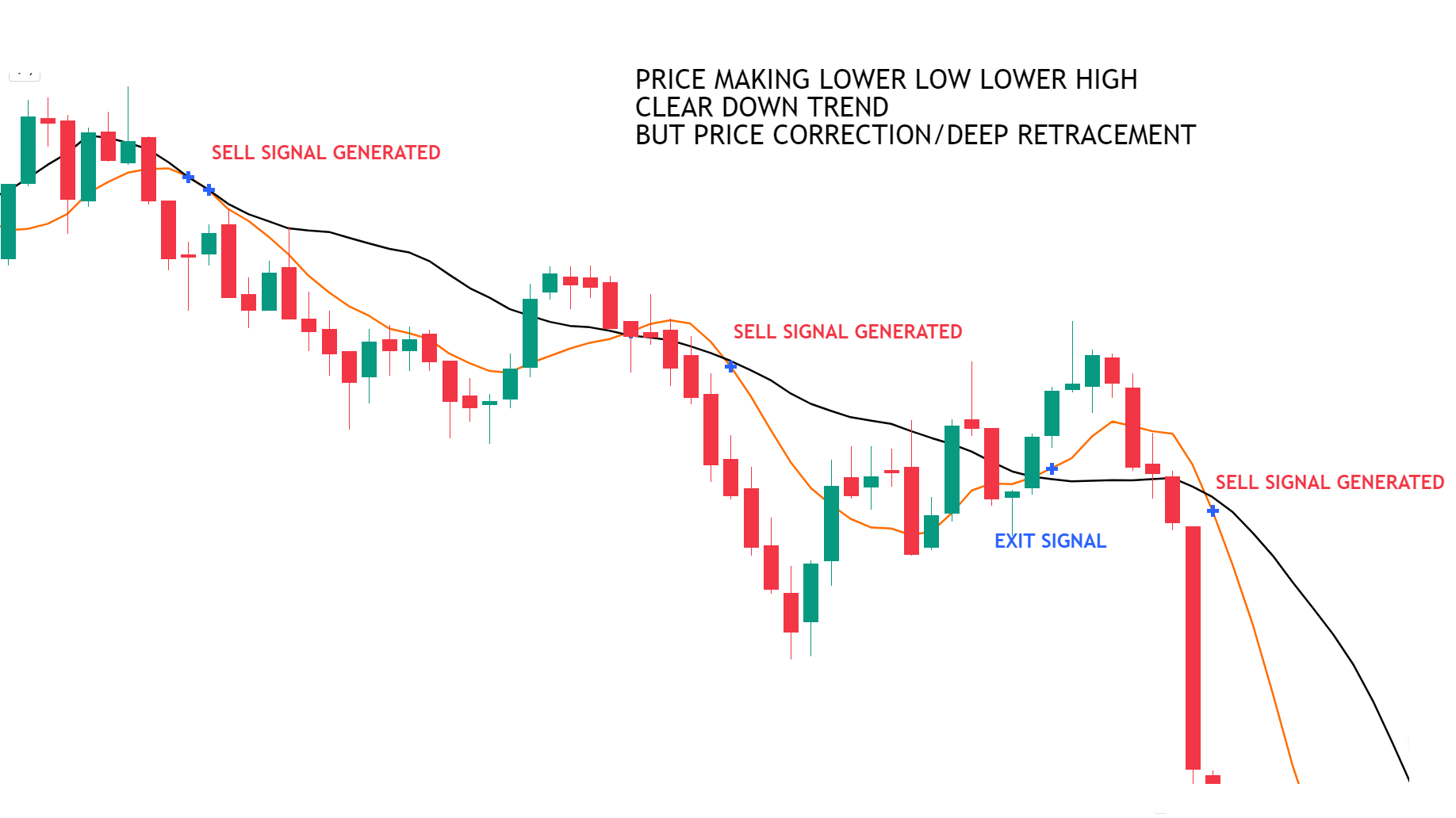Due to the rapid price movement and the late MA cross, the entry point was relatively late. The exit signal arrived far too late. And if the price gives deep retracement, then you will be out of trade irrespective of the price move in your direction. In this example, the deep retracement happens.

The Fibonacci method is being used by the other trader. He decides to trade swing, plots the Fibonacci retracement levels, and watches for an entrance signal at the level of retracement. Enter the deal as soon as the signal appears. In order to determine when to end the trade, he also draws the Fibonacci extensions level.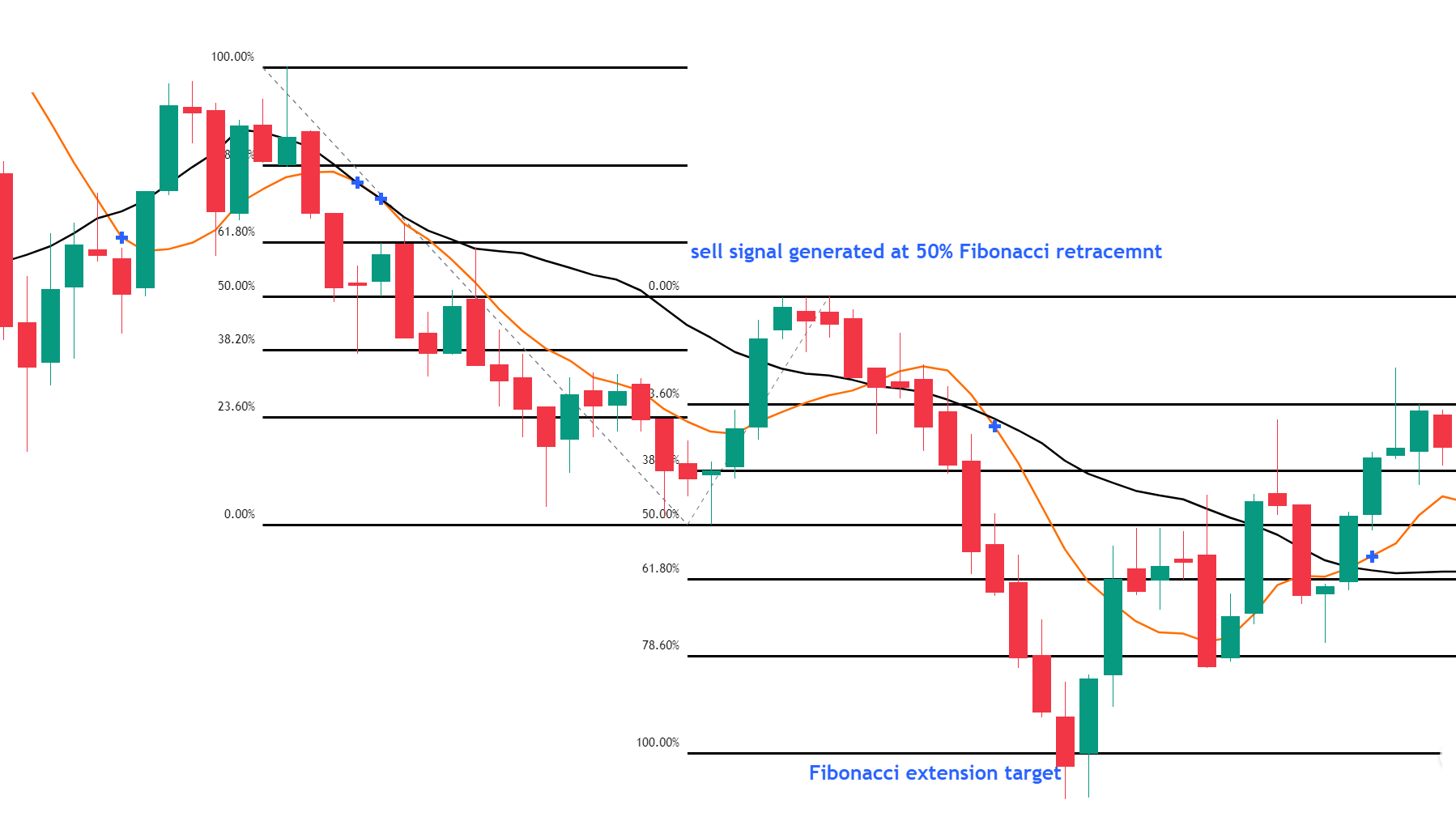When the moving average trend follows the system, the initial trader, decided to enter the trade. To make a profit, it was far too late. Examine the second trader (Fibonacci). Observe that he made his entry and exit decisions well before the first trader did. He increased his profit on the same chart and closed it out while the initial trader was still holding out for the trend to continue.

#### What Are the Different Fibonacci Trading Tools and How to Apply Them?

The Fibonacci tools are used to identify entries, support & resistance, targets, and exits. These two are the most widely used:

• Fibonacci retracement
• Fibonacci extension

#### What is a Fibonacci Retracement and how to use it?

The underlying principle of the Fibonacci retracement trading method. According to the theory, the price will retrace after starting a new trend direction before continuing in the trend’s direction. So, to determine the next potential support and resistance levels, we use the Fibonacci tool.

The retracement level predicts the highest level at which retracement is possible. These retracement levels offer traders a great chance to open new trades in the trend’s direction.

• The Fibonacci retracement levels are 23.6%, 38.2%, 50%,61.8%, 78.6%, and 100%.

Next possible support levels are marked if the stock moves up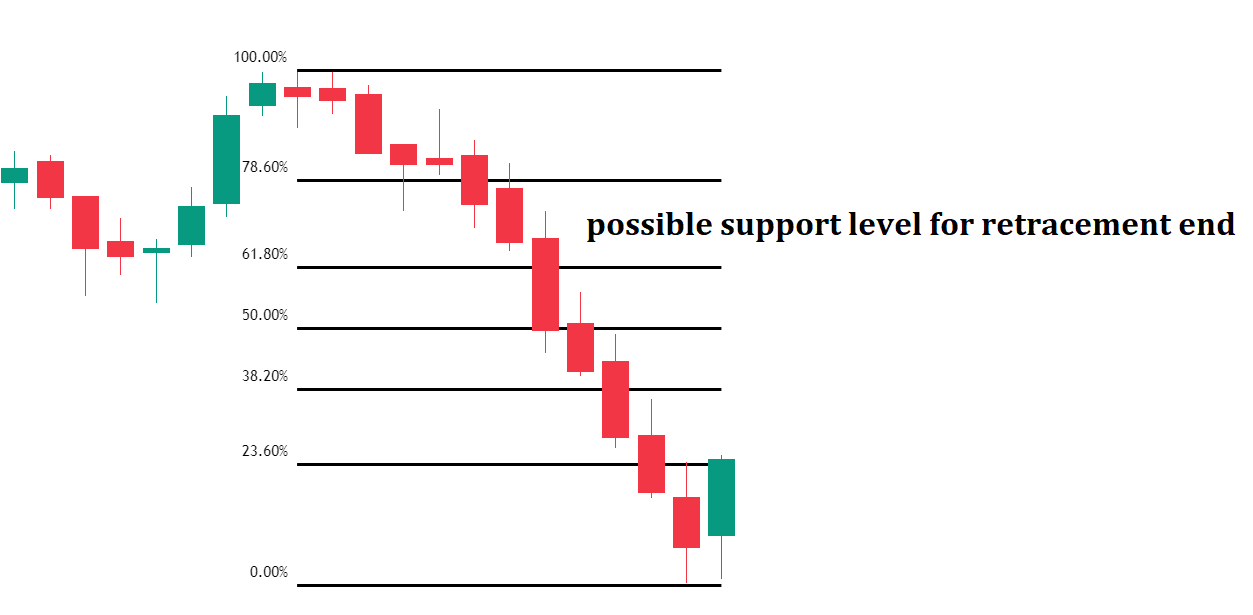####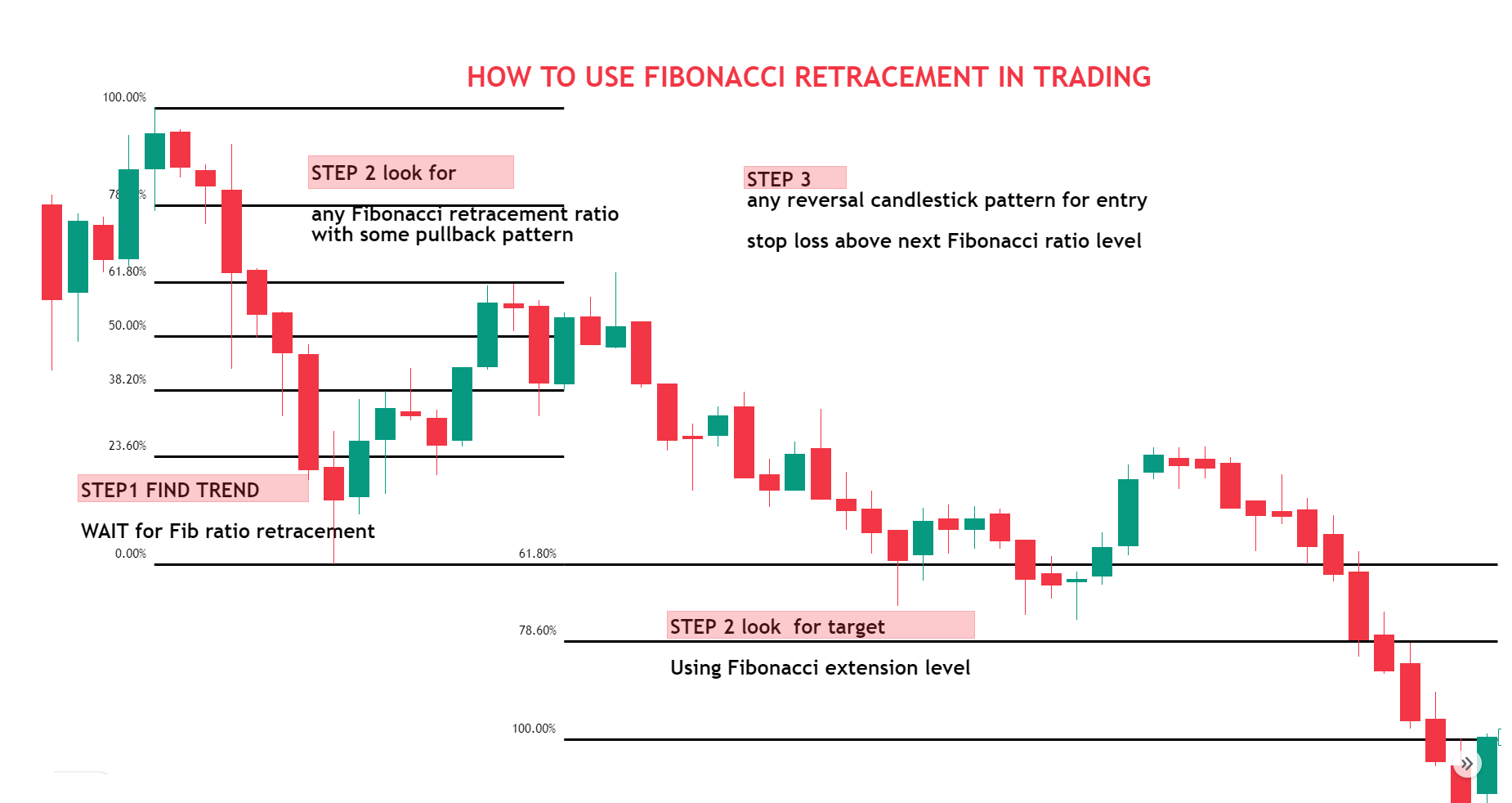How to draw Fibonacci Retracement?

EXAMPLE FIBONACCI RETRACEMENT IN A DOWNTREND

You must locate the most recent important Swing Highs and Swing Lows in order to determine these Fibonacci retracement levels. Find the recent swing HIGH (starting point) and recent swing LOW (ending point). then joint the 2 lines. Drag the pointer to the most recent Swing LOW to swing HIGH and find all retracement level

• 0.618 is the golden retracement level
• .0.5 to .618 is the imp retracement level in the trend
• Use the Fibonacci tool along with the confluence factor for entry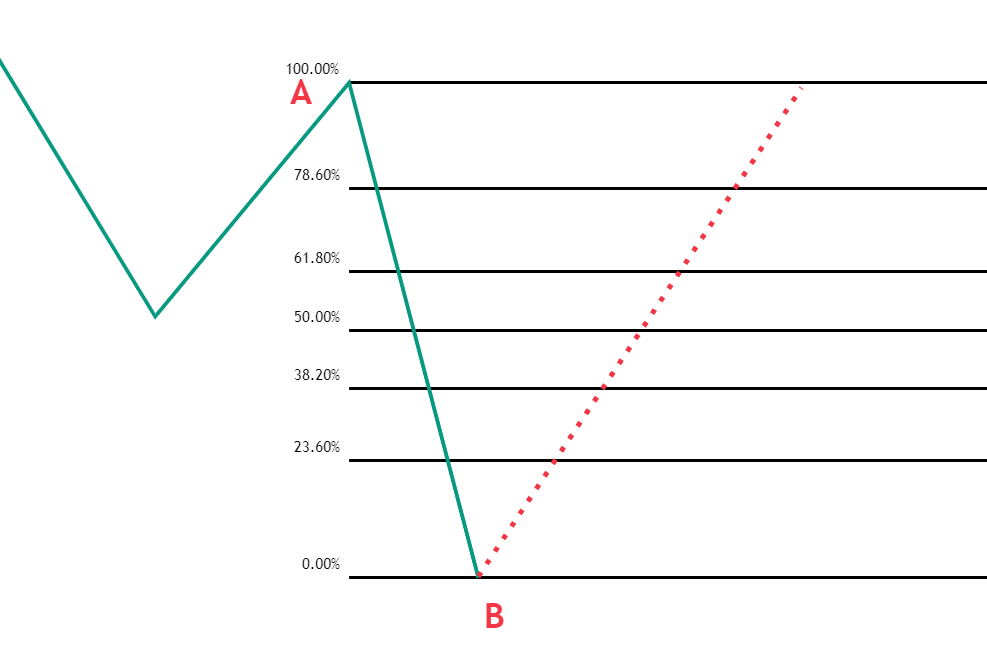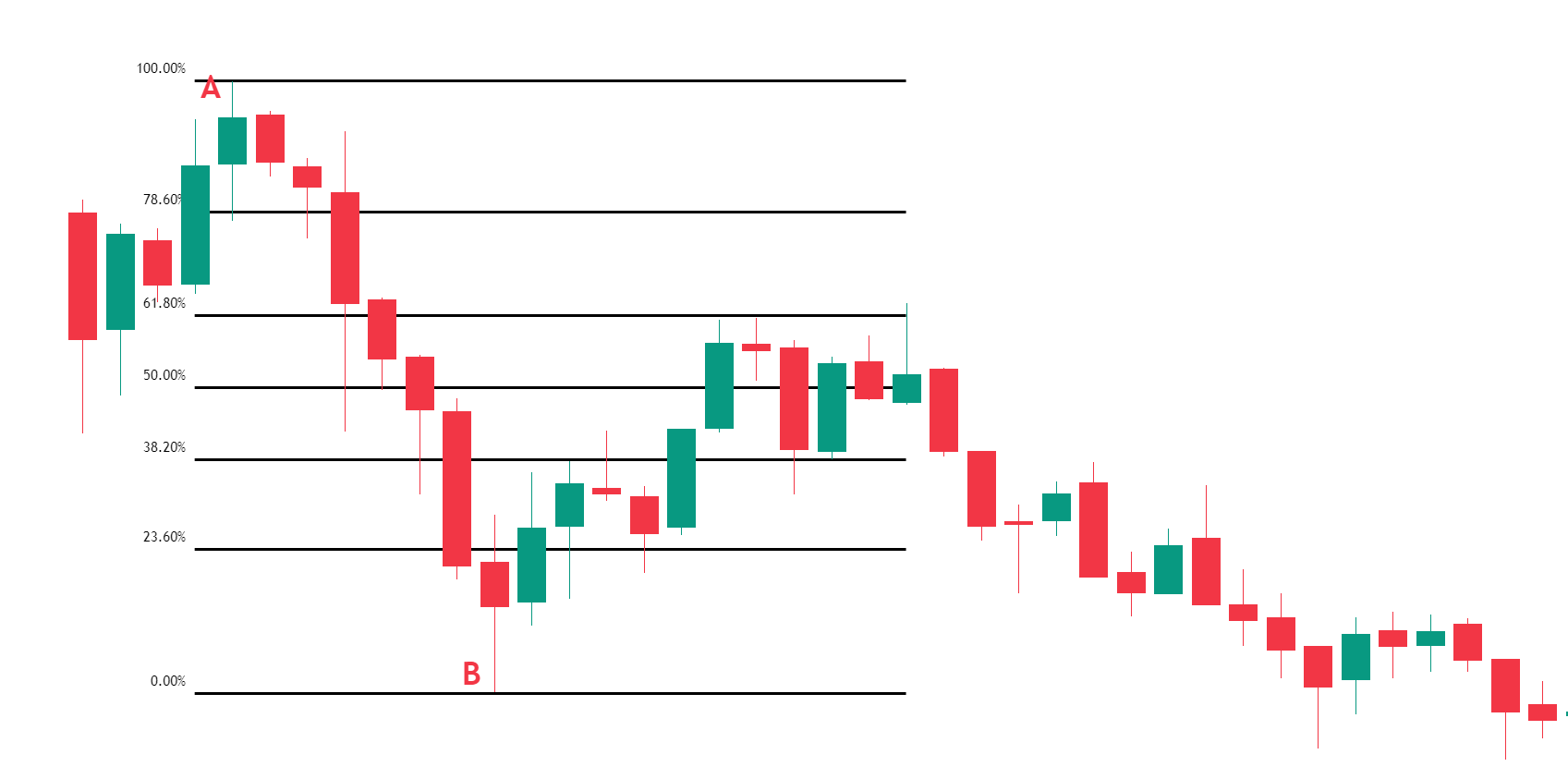Above chart price retrace 61.8% level. THE BELOW CHART is the continuation of the above chart. but retrace 50%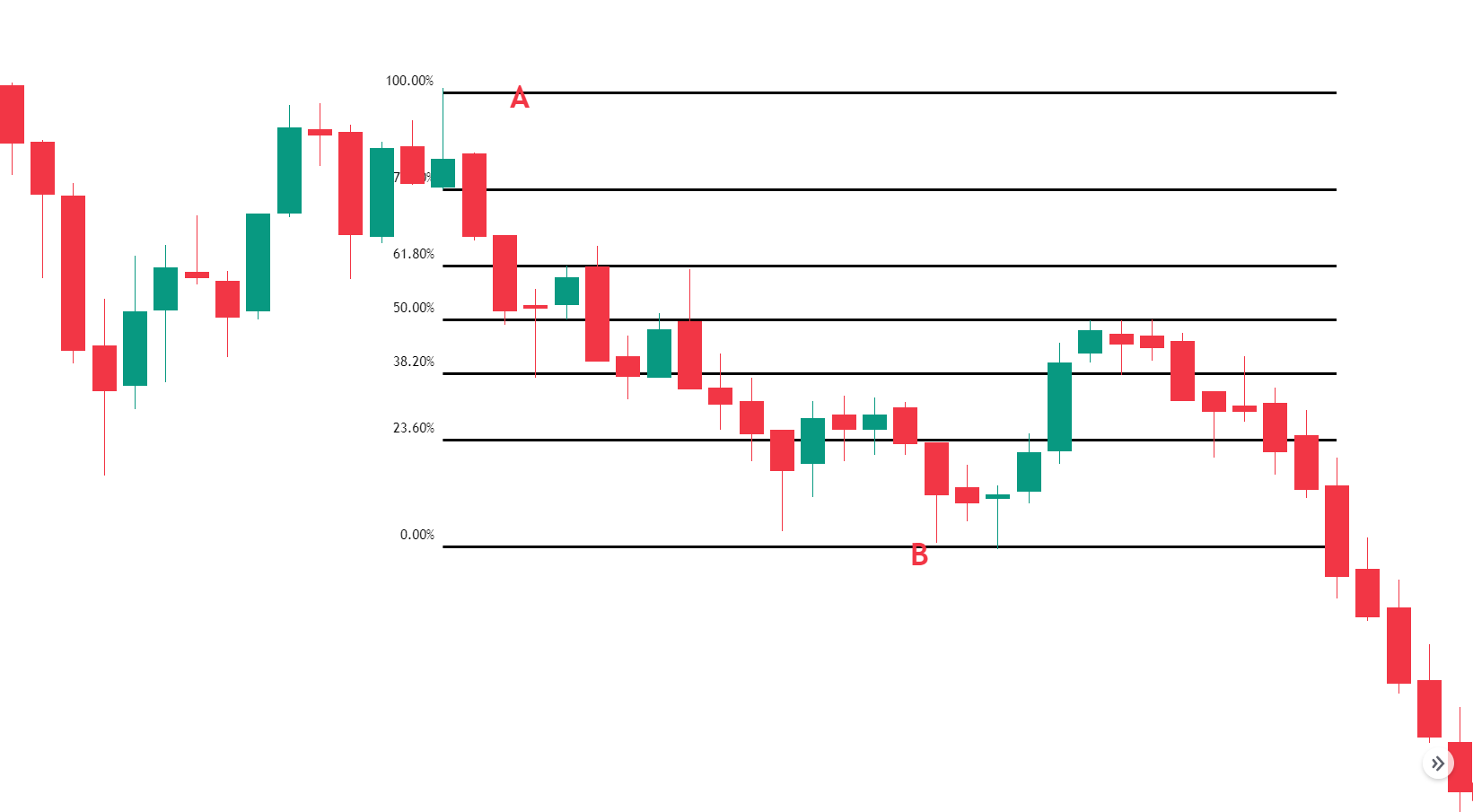Again, the below chart is the continuation of the above chart and retraces 78.6%

####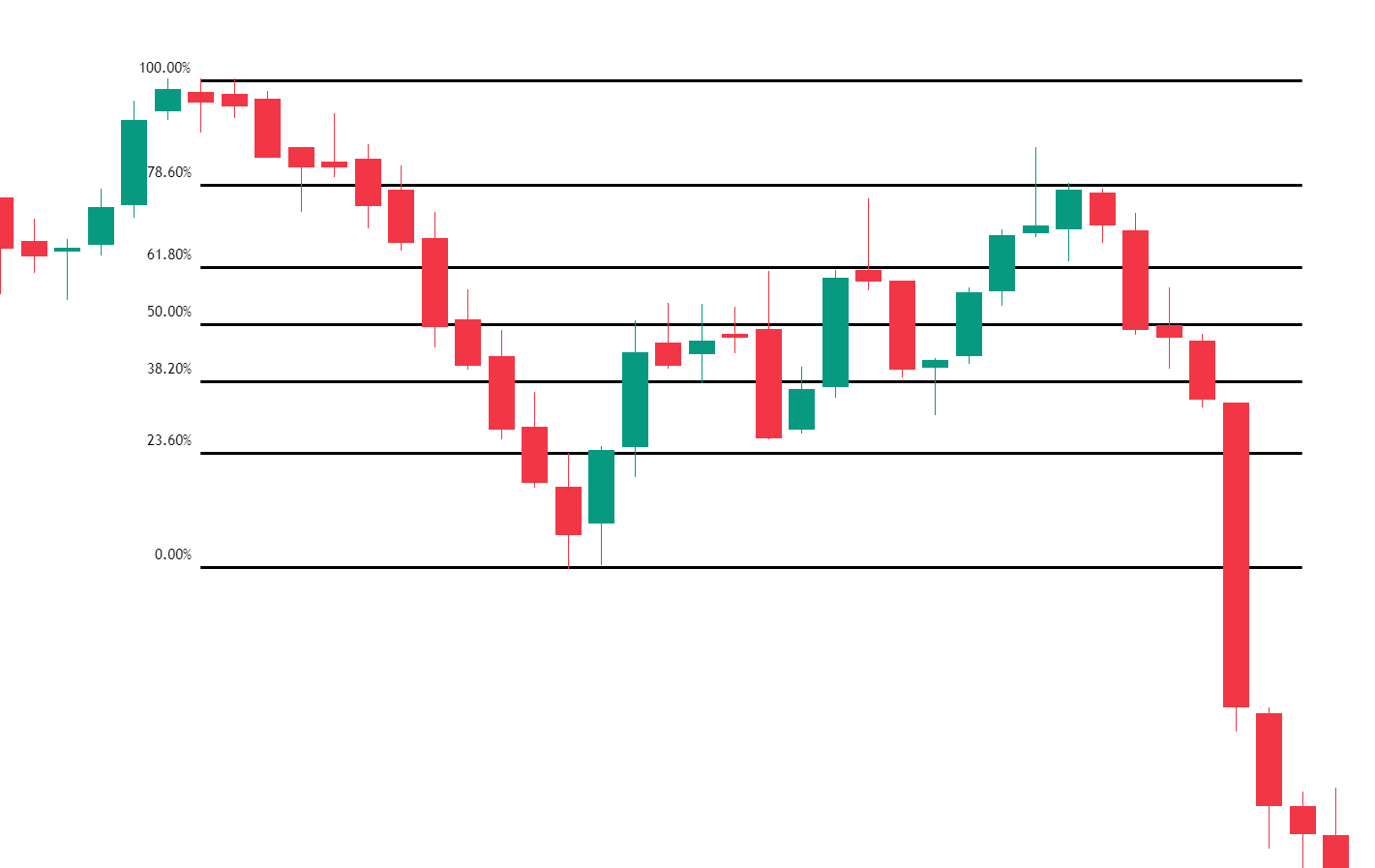What is the Fibonacci extension and how to use it? How to draw Fibonacci Extensions?

Fibonacci Expansion is based on three points. Here are the step for a downtrend

Step 1 – Identify the direction of the market: downtrend. To draw it we have to identify the impulse swing (A and B points) and retracement end (C point).

Step 2 – Attach the Fibonacci extension tool on the swing high and drag it to the right, all the way to the swing low.

Step 3 – Now, drag again back to the retracement end (point C)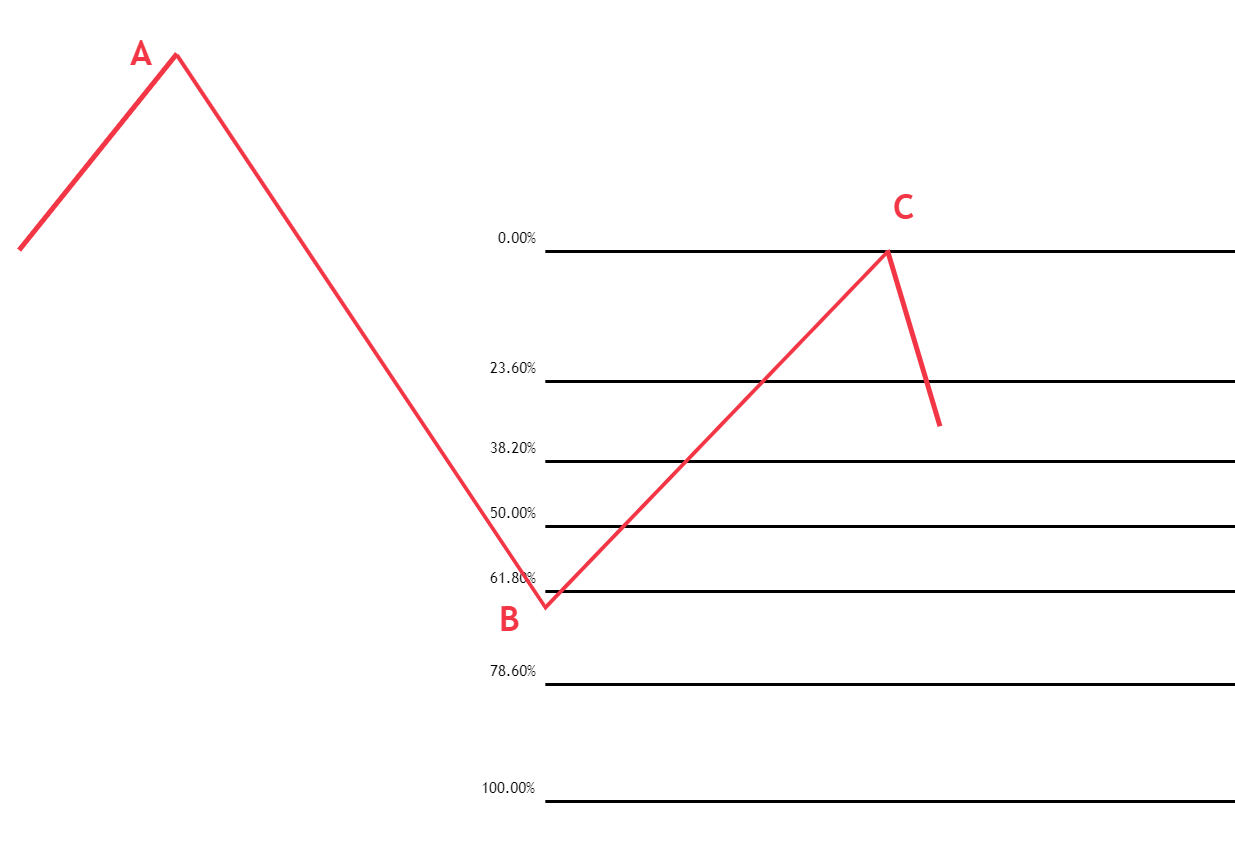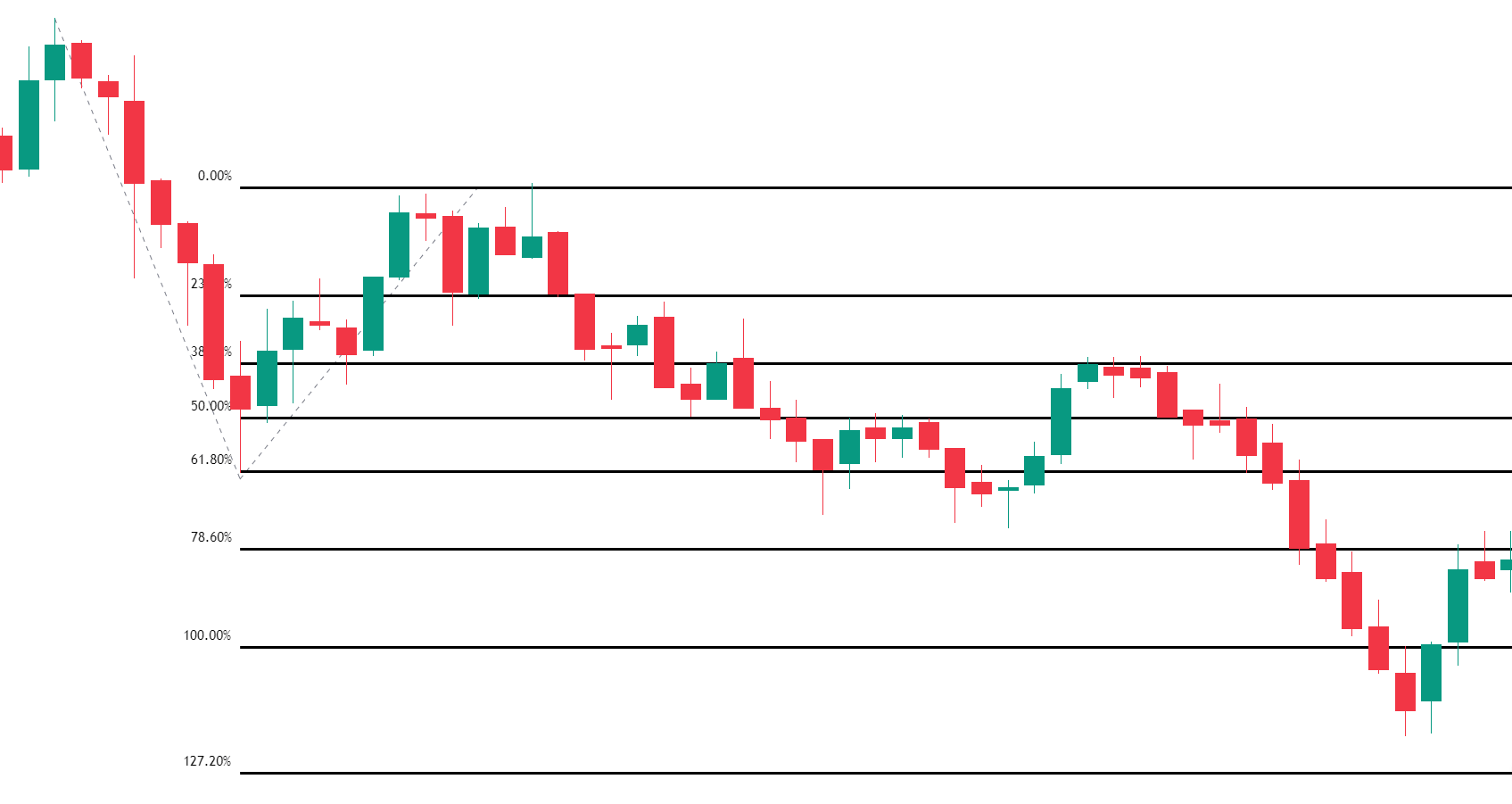#### Why use Fibonacci Extensions in Trading?

Opening a trade is significantly less significant than closing it. Extension tool for exit price goal. Traders can use Fibonacci extensions as a tool to set profit targets or predict how far a market may rise when a retracement is complete. Extension levels are yet another potential location for a price reversal.

Fibonacci extension levels are quite helpful in deciphering market reversals and potential roadblocks. Simply put, Fibonacci extension levels are the critical points from which the price of an instrument may change.

The Fibonacci Retracement Tool makes it easy to lay out extensions by automatically identifying several extension levels where prices can turn around. Common Fibonacci extension levels are 61.8%, 100%, 161.8%, 200%, and 261.8%.

In order to fully exit a trade or book a partial profit, traders must adhere to certain guidelines. They are aware that there is a good likelihood the movement will come to an end or pause for a while.

Divide your position into three parts, which is the basic profit booking strategy traders use. At 100% extension, the first part is immediately closed. You close the second part at the 161.8% extension if the price continues to move in the direction of the trend. You let the third component increase before manually closing it using either a technical trigger or an extension level that is different.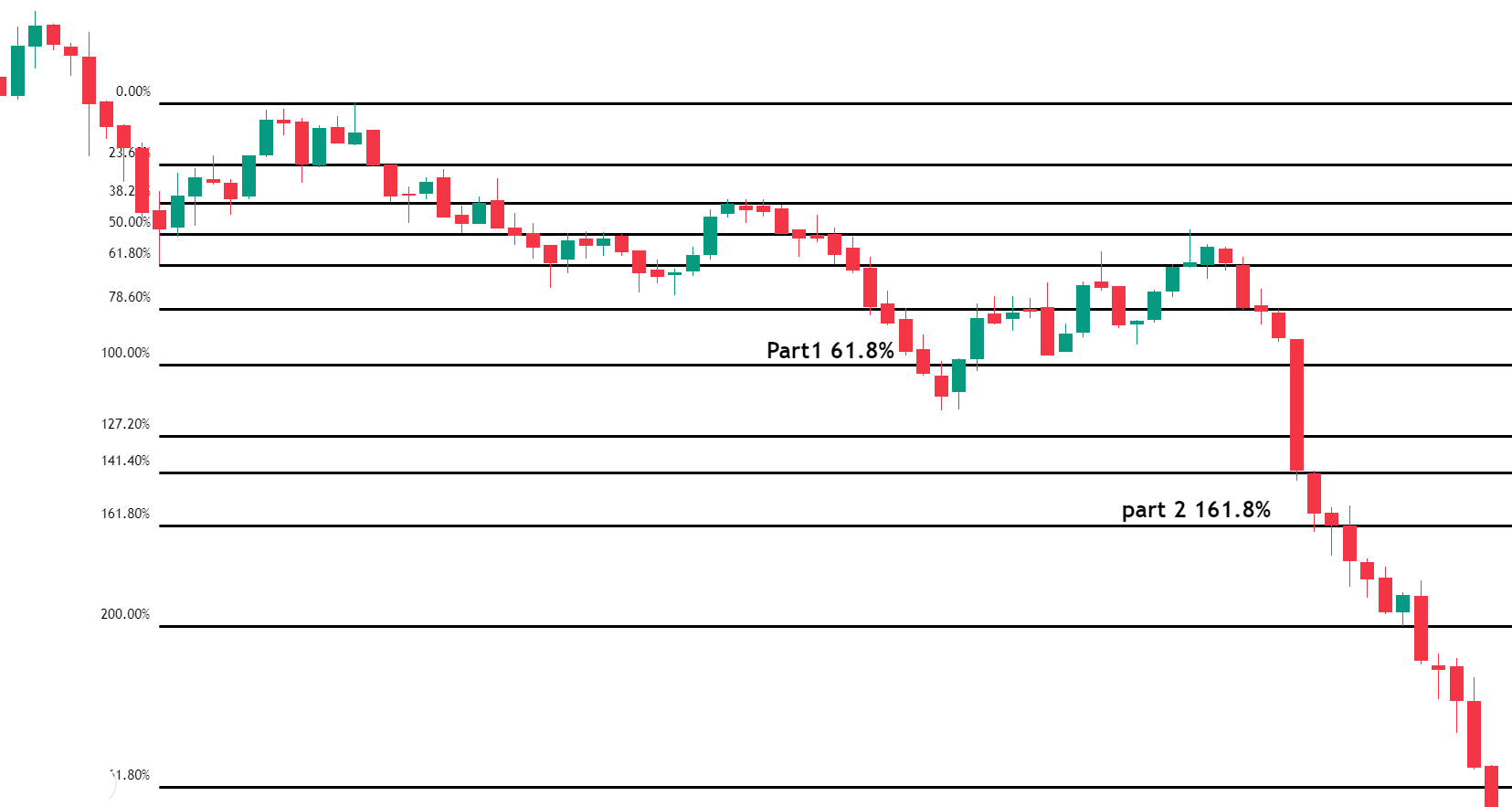#### Difference Between Fibonacci Retracement and Fibonacci Extension

 Fibonacci Retracement Fibonacci Extension Indicates how depth of retracement after impulse move. Indicates where the price may go following a retracement in the trend Measures Pullbacks Within a Trend. price correction or time correction Measures the trend impulses waves in the direction of the trend Provide effective stop-loss levels and entry orders for a trend trading strategy. Gives good take-profit points in trend trading and reversal points for trend reversal techniques. With other confluences, it can be effectively used as a profitable approach. It can be applied to a strategy for taking profits and may also indicate promising points for trend reversals. Fibonacci Numbers are within the initial trend. (38.2%, 61.8%, 50%, etc.) Fibonacci Numbers are beyond the 100% Fibonacci level. (1.618%, 123.60%, etc.)The Fibonacci technique works best when the market is trending, which is the first thing you should know about it. When the market is moving upward, the plan is to buy a retracement at a Fibonacci support level. Additionally, when the market is trending downward, it is advisable to sell a retracement at a Fibonacci resistance level. Since Fibonacci retracement levels try to foretell where the price might be in the future, they are regarded as a type of technical indicator.

• What Fibonacci retracement levels should I use?
• Should the price touch the retracement levels?
• What confluence factor to check for more reliable entry?
• How to take entry (aggressive /conservative/safe entry)?
• Where to put stop loss based on entry?
• Where to take entry?

#### What Fibonacci Retracement Levels Should I use?

Because there are so many Fibonacci retracement levels, it is highly confusing at first. Fibonacci Retracements are not entry signals, they are target areas where an entry signal may occur. Trading simply because the price has reached a Fibonacci retracement ratio level is not a wise strategy. Another confluence is required before trades can be taken.

• If u use Fibonacci Retracements level with other concepts confirming like trend line, pivot point, or any dynamic support or resistance

#### Should the price touch the retracement levels?

This is always a problem for beginners. They think that the retracement to any fibo level is only valid when the price touches down this level. They are wrong. Fibonacci retracements are a great tool, but there is no 100% accuracy. Sometimes the price closes near the retracement level and it can be still a valid move.

#### How to take entry?

Basically, 3 types of entry can be taken after a Fibonacci retracement.

• Aggressive entry
• Conservative entry
• Safe entry

Which entry is the best for you? It is your decision. It depends on risk, reward, and probability.

#### Aggressive entry after deep retracement

This is the riskiest entry but a small loss and high reward setup. You are willing to take a small risk in turn for a possible bigger return. When the price near/reaches the 61.8% retracement, you go long at this level or a little bit above it.

1. Identify the trend and level of Fibonacci retracement level.
2. Look for the confluence factor
3. Wait for a reversal candlestick pattern for entry
4. Draw extension lines for the possible target level
5. Put stop loss beyond recent high in downtrend also beyond Fibonacci retracement level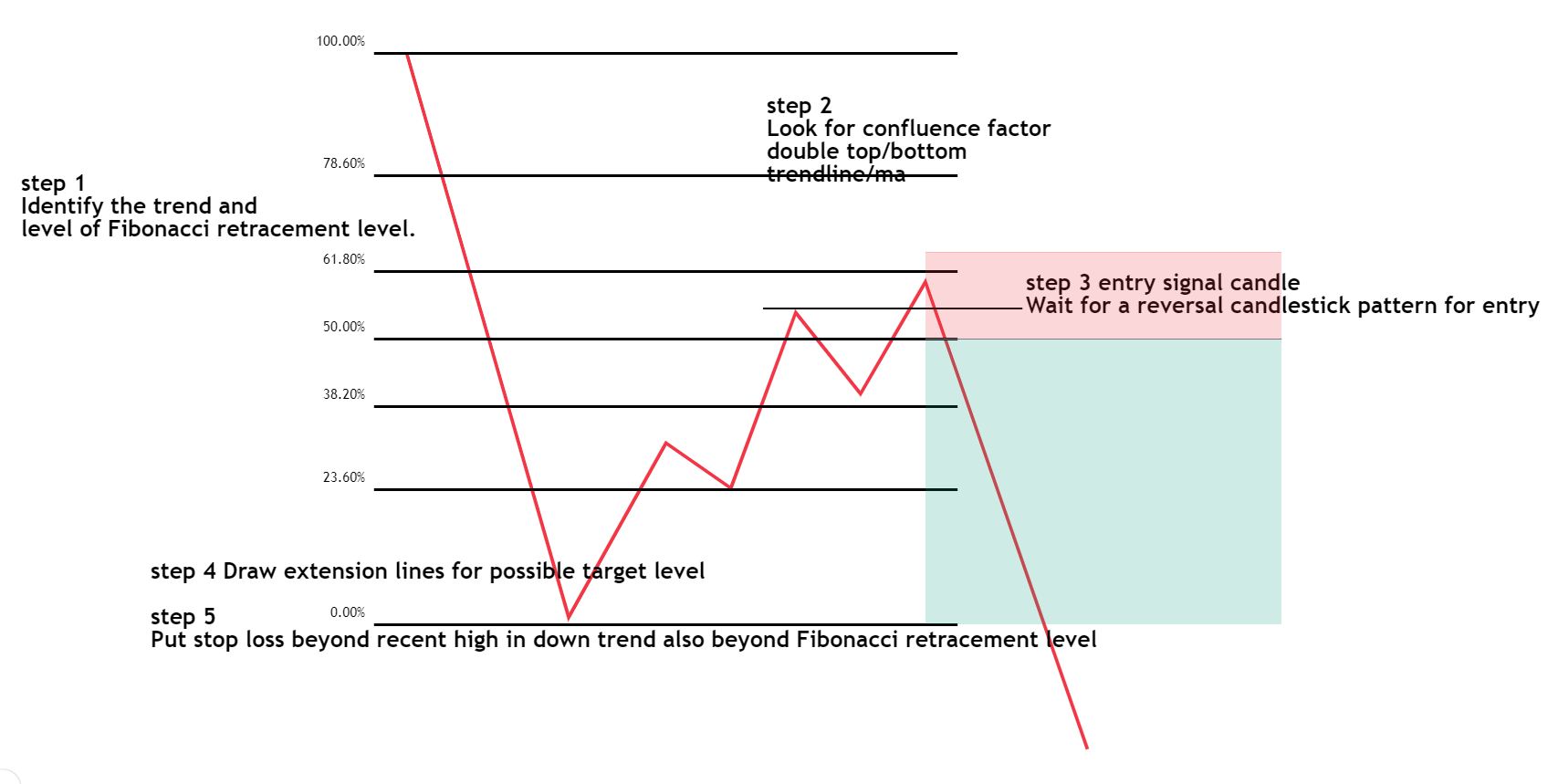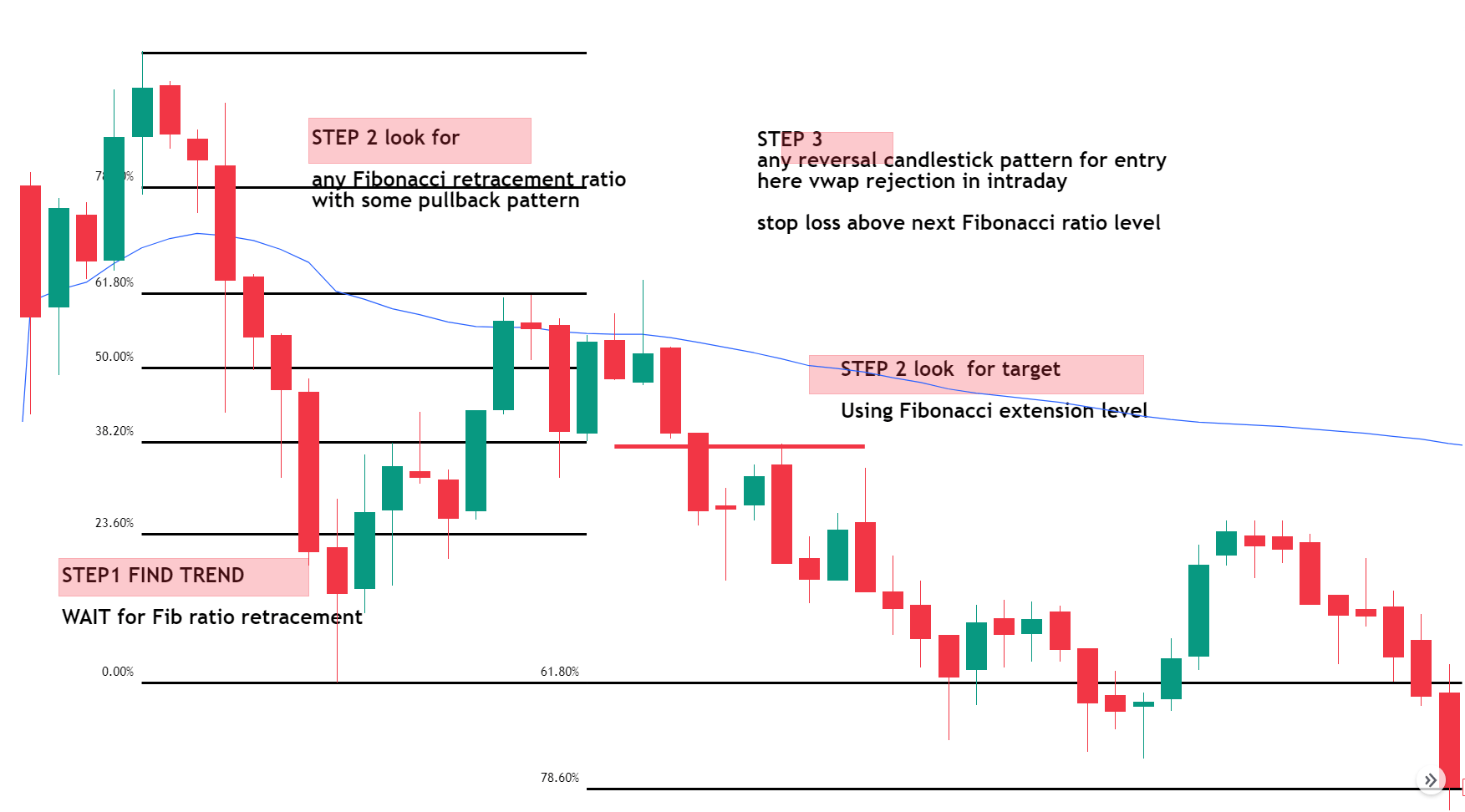#### Conservative Entry

The conservative entry is when you wait and watch how the price reacts toward the retracement levels. If you see that 61.8% is probably the retracement which a bounce back may occur from, you are ready to take a long position. But unlike the aggressive entry case, you wait for another confirmation. Break of minor swing low in the bearish entry. confirmation signals are not always 100% correct, but in that case, you have a lower chance of failure. The ratio between risk and possible profit is good. In this example, the trader decided that the signal will be close below support.

1. Identify the trend and level of Fibonacci retracement level.
2. Look for the confluence factor
3. Wait for the break of minor support for entry for a bearish trade
4. Draw extension lines for the possible target level
5. Put stop loss beyond recent high in downtrend also beyond Fibonacci retracement level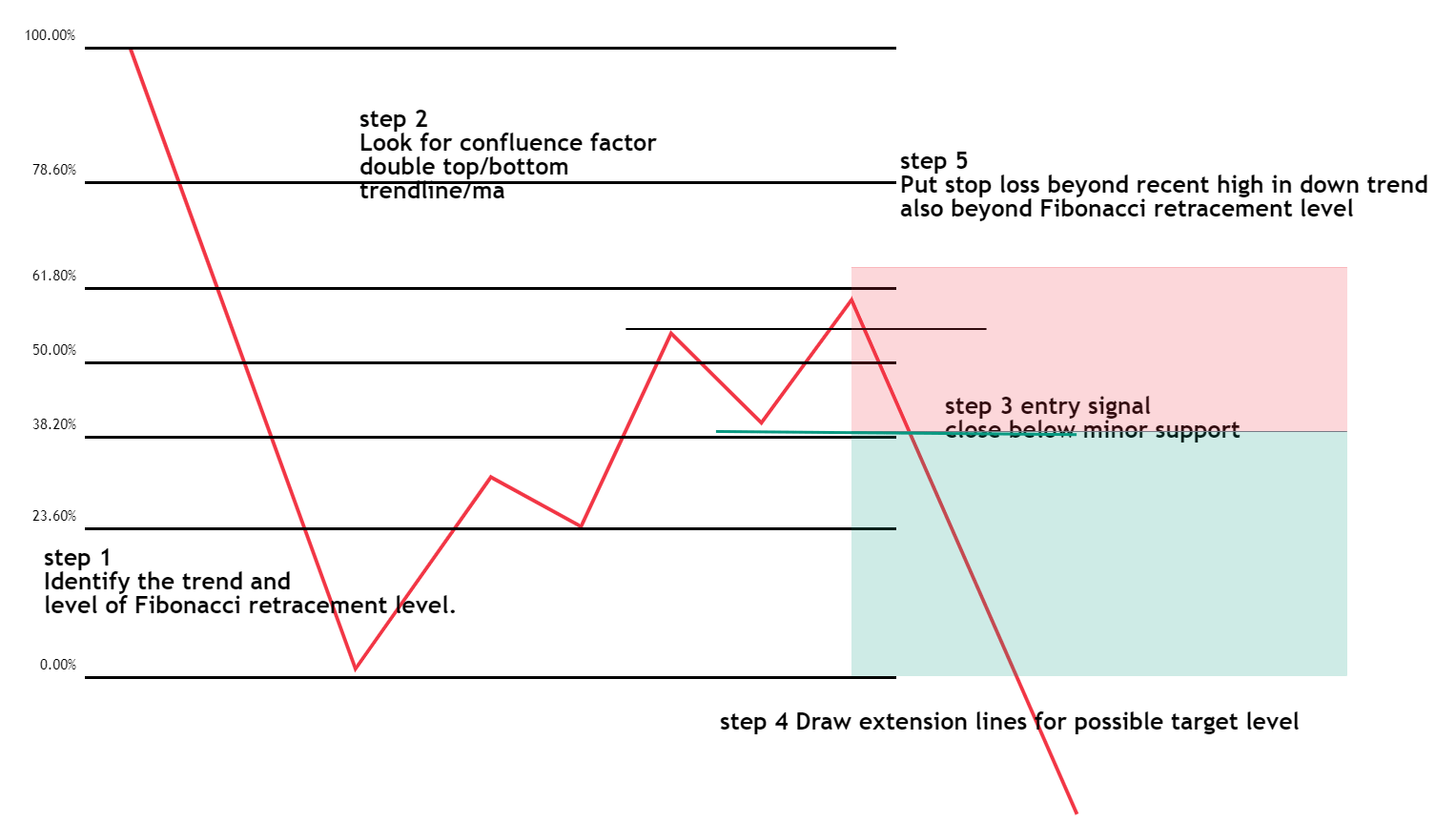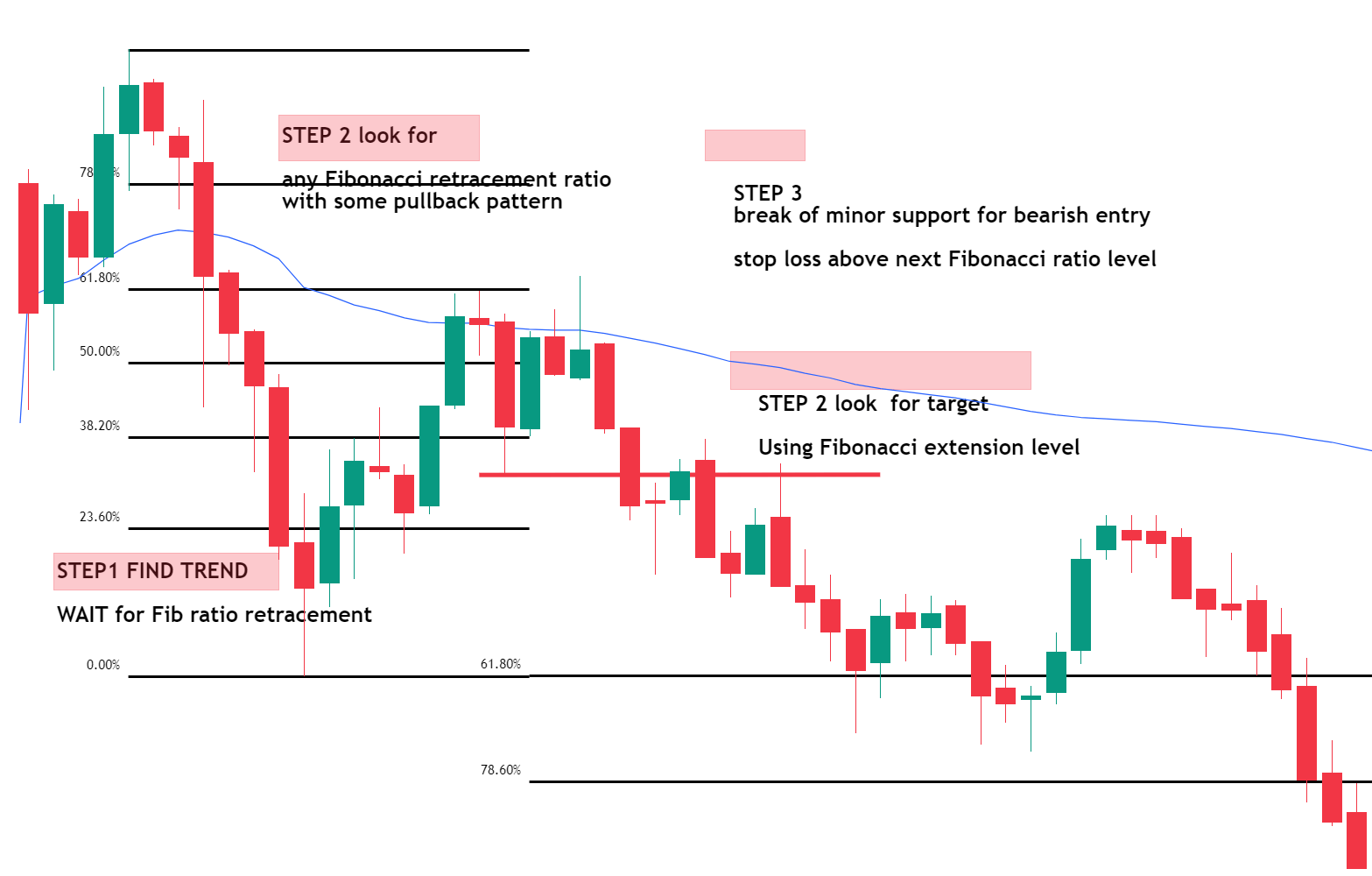One more example of both entry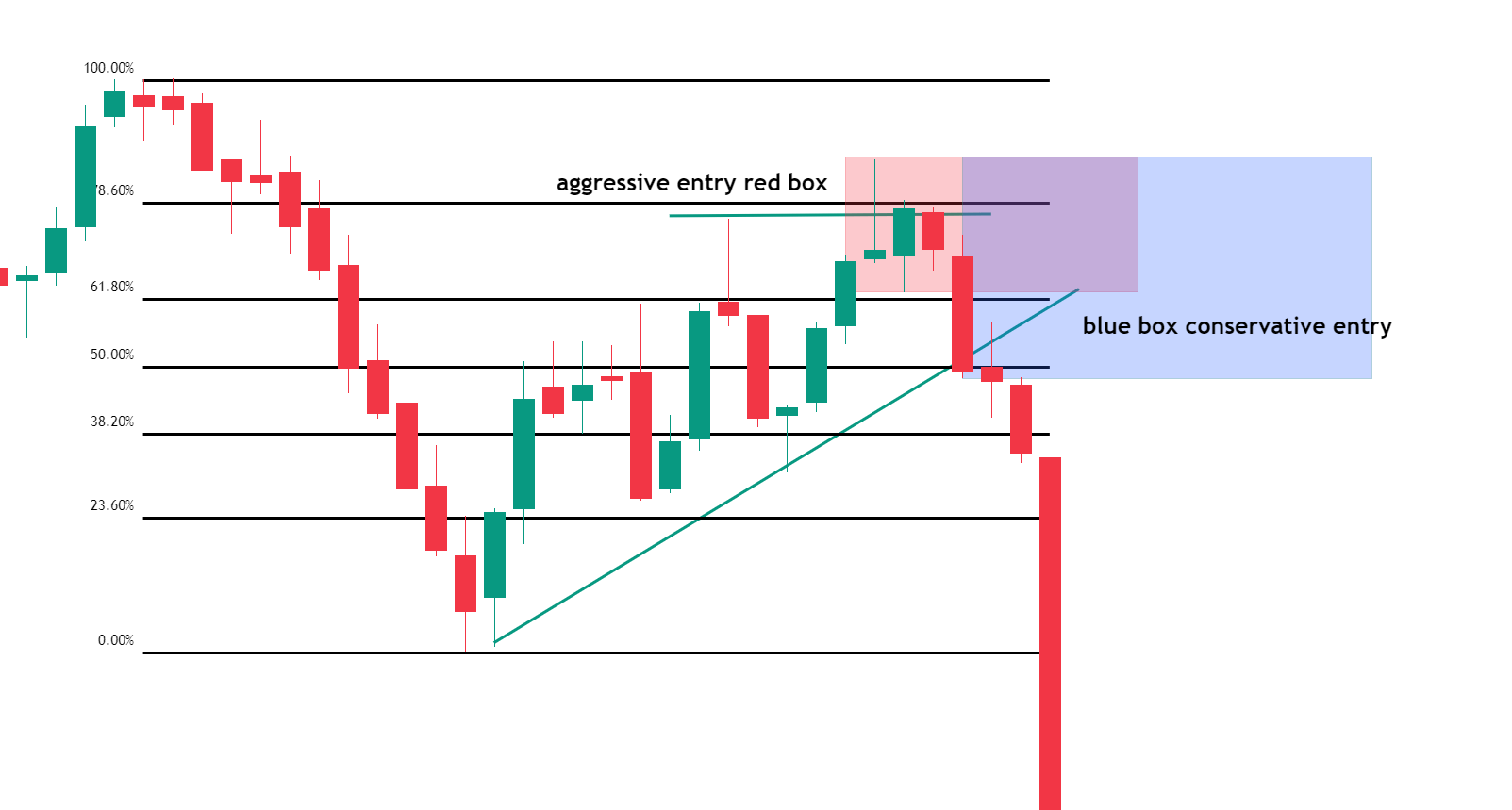#### Safe Entry

This way of trading is the safest one compare to another 2 entry methods, but your possible profit is the smallest. The main idea is to buy a breakout after a price contraction.

In theory, it should look like in the picture below:

1. Identify the trend and level of Fibonacci retracement level.
2. Look for price contraction before the breakout
3. Wait for the break of support for entry for a bearish trade
4. Draw extension lines for the possible target level
5. Put stop loss beyond contraction high in a downtrend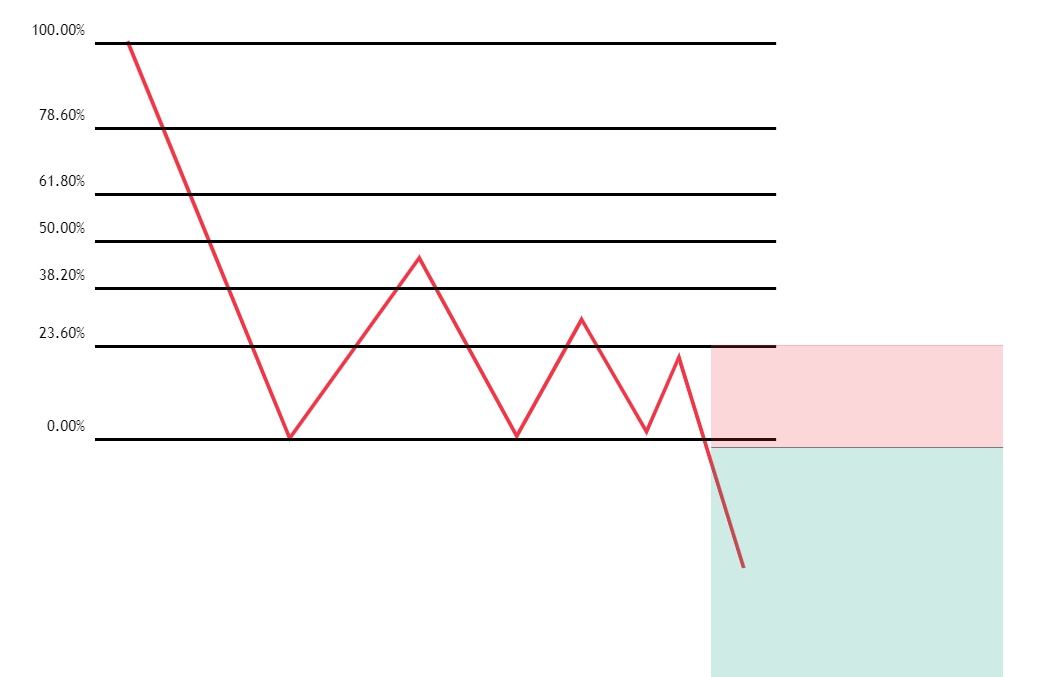In the next section, we will discuss the following Fibonacci confluence strategies in detail.

• Fibonacci with support resistance entry method
• Fibonacci with trendline entry method
• Fibonacci with moving average entry method
• Fibonacci with price pattern entry method
• The Fibonacci convergence entry method

1.2.